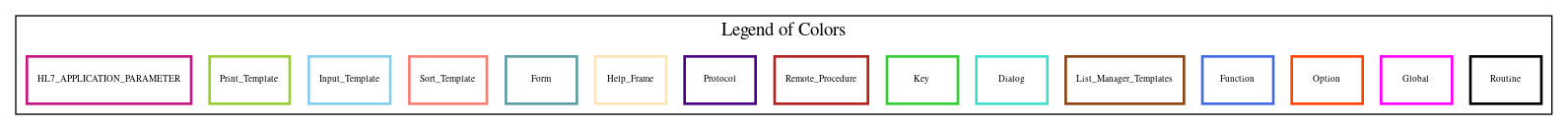Home   Package List   Routine Alphabetical List   Global Alphabetical List   FileMan Files List   FileMan Sub-Files List   Package Component Lists   Package-Namespace Mapping
Routine: IBCEOB0

# Routine: IBCEOB0

## Information

IBCEOB0 ;ALB/TMP/PJH - 835 EDI EOB MSG PROCESSING ; 8/24/10 7:23pm

## Source Information

Source file <IBCEOB0.m>

## Call Graph### Call Graph Total: 8

Package Total Call Graph
Integrated Billing 5 \$\$DOLLAR^IBCEOB  (DET40,DET4X)^IBCEOB00  (\$\$FINDLN,\$\$STORE)^IBCEOB1  \$\$DATE^IBCEU  \$\$GETSPEC^IBEFUNC
VA FileMan 3 FILE^DICN  ^DIE  \$\$GET1^DIQ

## Caller Graph

### Legends:#### Package Component Superscript legend

 action A extended action Ea event driver Ed subscriber Su protocol O limited protocol LP run routine RR broker B edit E server Se print P screenman SM inquire I### Caller Graph Total: 1

Package Total Caller Graph
Integrated Billing 1 IBCEOB

## Entry Points

LINE() ;Extract Provider Line Reference from 42 record
30(IB0,IBEOB,IBOK) ; Process record type 30 for EOB
; IB0 = the record being processed
; IBEOB = the ien of the EOB entry in file 361.1
; IBOK = Returned as 1 if record filed OK, 0 if error occurred
Q30
40(IB0,IBEOB,IBOK) ; Process record type 40 for EOB
; IB0 = the record being processed
; IBEOB = the ien of the EOB entry in file 361.1
; IBOK = Returned as 1 if record filed OK, 0 if error occurred
Q40
41(IB0,IBEOB,IBOK) ; Process record type 41 for EOB
; IB0 = the record being processed
; IBEOB = the ien of the EOB entry in file 361.1
; IBOK = Returned as 1 if record filed OK, 0 if error occurred
Q41
42(IB0,IBEOB,IBOK) ; Process record type 42 for EOB
; IB0 = the record being processed
; IBEOB = the ien of the EOB entry in file 361.1
; IBOK = Returned as 1 if record filed OK, 0 if error occurred
Q42
45(IB0,IBEOB,IBOK) ; Process record type 45 for EOB
; IB0 = the record being processed
; IBEOB = the ien of the EOB entry in file 361.1
; IBOK = Returned as 1 if record filed OK, 0 if error occurred
Q45
46(IB0,IBEOB,IBOK) ; Process record type 46 for EOB
; IB0 = the record being processed
; IBEOB = the ien of the EOB entry in file 361.1
; IBOK = Returned as 1 if record filed OK, 0 if error occurred
Q46
ERRTXT(X,IBEOB) ; Set error text based on circumstances
; Input - X = Standard Error message passed in
; IB0
; Returns modified error message text
EBILL(IBEOB) ; Check If EOB was billed electronically
; Input : IBEOB = Internal entry number from file 361.1
; Returns : 1 - Billed electronically
; 0 - Not billed electronically

## External References

Name Field # of Occurrence
FILE^DICN 40+38, 40+51, 42+18, 45+23, 45+32, 46+24
^DIE 41+16
\$\$GET1^DIQ EBILL+5, EBILL+9
\$\$DOLLAR^IBCEOB 41+14, 41+15
DET40^IBCEOB00 40+32
DET4X^IBCEOB00 41+8, 42+9, 45+8, 46+9
\$\$FINDLN^IBCEOB1 40+22
\$\$STORE^IBCEOB1 30+8, 40+46, 45+40
\$\$DATE^IBCEU 40+37
\$\$GETSPEC^IBEFUNC 45+21, 45+30, 46+22

## FileMan Files Accessed Via FileMan Db Call

FileNo Call Tags
^IBM(361.1 - [#361.1] GET1^DIQ
^IBA(364 - [#364] GET1^DIQ

## Global Variables Directly Accessed

Name Line Occurrences  (* Changed,  ! Killed)
^IBA(364 - [#364] EBILL+6
^IBM(361.1 - [#361.1] 40+10, 40+11, 40+41, 41+25, 42+15, 42+26, 45+16, 46+16
^TMP( 30+9*, 40+12*, 40+27*, 40+30*, 40+31*, 40+33*, 40+39*, 40+47*, 40+53*, 41+7*
, 41+9*, 41+17*, 41+32*, 42+8*, 42+10*, 42+20*, 42+32*, 45+7*, 45+9*, 45+13*
, 45+24*, 45+33*, 45+41*, 46+8*, 46+13*, 46+25*
^TMP(\$J 40+8!, 40+46*, 41+6, 41+12, 41+29, 41+31*, 41+32, 42+7, 42+14, 42+29
, 42+31*, 42+32, 45+6, 45+11*, 45+12, 45+15, 45+24, 45+33, 45+41, 46+7
, 46+12, 46+15, 46+25

## Label References

Name Line Occurrences
\$\$EBILL ERRTXT+6
\$\$ERRTXT 40+30
\$\$LINE 40+20
45 41+30, 42+30
Q40 40+12, 40+23, 40+39, 40+47, 40+53
Q41 41+6
Q42 42+7
Q45 45+6, 45+13
Q46 46+7, 46+13

## Local Variables

### Legend:

 >> Not killed explicitly * Changed ! Killed ~ Newed

Name Field # of Occurrence
A 30+5~, 30+6*, 30+8, 40+7~, 40+44*, 40+45*, 40+46, 45+5~, 45+39*, 45+40
, 46+6~
AGC 46+6~, 46+12*, 46+13, 46+16
CT 40+24~, 40+33*, 41+5~, 41+9*, 42+5~, 42+10*, 45+5~, 45+9*, 46+6~
DA 40+7~, 40+52!, 41+5~, 41+12*, 42+5~, 45+19~
DA(1 40+35*, 40+50*, 41+12*, 41+13, 42+14*, 42+15, 45+20*, 45+29*, 46+21*
DA(2 40+50*, 42+14*, 42+15, 45+20*, 45+29*, 46+21*
DA(3 45+29*, 46+21*
DD 40+7~, 40+38!, 40+51!, 42+5~, 42+12!, 42+18!, 45+19~, 45+23!, 45+32!, 46+24!
DIC 40+7~, 40+35*, 40+38!, 40+50*, 40+52!, 42+5~, 42+15*, 45+19~, 45+20*, 45+23!
, 45+29*, 45+32!, 46+21*, 46+24!
DIC("DR" 40+37*, 42+16*, 42+17*
DIC("P" 45+21*, 45+30*, 46+22*
DIC(0 40+35*, 40+50*, 42+15*, 45+20*, 45+29*, 46+21*
DIE 41+5~, 41+13*
DLAYGO 40+7~, 40+35*, 40+38!, 40+50*, 40+52!, 42+5~, 42+12!, 42+15*, 42+18!, 45+19~
, 45+20*, 45+23!, 45+29*, 45+32!, 46+21*, 46+24!
DO 40+7~, 40+38!, 40+51!, 42+5~, 42+12!, 42+18!, 45+19~, 45+23!, 45+32!, 46+24!
DR 40+52!, 41+5~, 41+11*, 41+14*, 41+15*, 41+16
>> EFLAG 40+25*
ERRCOD 40+7~, 40+21*, 40+22, 40+28, 40+32
ETEXT 40+24~, 40+25*, 40+28*, 40+29*, 40+30
>> HIPAA 40+20
IB0 30~, 30+8, 40~, 40+15*, 40+16*, 40+17*, 40+20, 40+22, 40+32, 40+37
, 40+45, 40+46, 40+51, 41~, 41+8, 41+14, 41+15, 41+25, 41+27, 42~
, 42+9, 42+16, 42+17, 42+26, 42+28, 45~, 45+8, 45+11, 45+12*, 45+13
, 45+16, 45+22, 45+24, 45+31, 45+33, 45+40, 45+41, 46~, 46+9, 46+23
, 46+25
IB45 41+26~, 41+27*, 41+28*, 41+30, 42+27~, 42+28*, 42+30
>> IBA1 LINE+2
IBDA 40+7~, 45+5~, 45+24!, 45+33!, 45+34*, 45+36, 45+38, 46+6~, 46+25!, 46+26*
IBDA(1 45+16*, 45+18, 45+25*, 45+28, 45+29, 45+37, 45+38, 46+16*, 46+20, 46+21
IBDA(2 45+15*, 45+16, 45+20, 45+29, 45+37, 45+38, 46+15*, 46+16, 46+21
>> IBEGBL 30+9, 40+12, 40+27, 40+30, 40+31, 40+33, 40+39, 40+47, 40+53, 41+7
, 41+9, 41+17, 41+32, 42+8, 42+10, 42+20, 42+32, 45+7, 45+9, 45+13
, 45+24, 45+33, 45+41, 46+8, 46+13, 46+25
IBEOB 30~, 30+8, 40~, 40+10, 40+11, 40+22, 40+30, 40+35, 40+41, 40+43
, 40+46, 40+50, 41~, 41+12, 41+25, 41+30, 42~, 42+14, 42+26, 42+30
, 45~, 45+16, 45+20, 45+29, 45+37, 45+38, 45+40, 46~, 46+16, 46+21
, ERRTXT~, ERRTXT+6, EBILL~, EBILL+5
>> IBFILE LINE+5, LINE+6
IBIFN 40+7~, 40+10*
IBLREF 40+7~, 40+22*, 40+23, 40+37
IBOK 30~, 30+8*, 30+9, 40~, 40+46*, 40+47, 40+51*, 40+53, 41~, 41+11*
, 41+16*, 41+17, 41+30, 41+32, 42~, 42+6*, 42+13*, 42+19*, 42+20, 42+30
, 42+32, 45~, 45+40*, 45+41, 46~, 46+5*, 46+26*
IBPC 40+7~
IBSAV40 41+26~, 41+29*, 41+31, 42+27~, 42+29*, 42+31
IBSEQ 40+7~, 40+11*, 40+12, 40+36, 40+39, 40+47, 40+53
>> IBZDATA 40+22
IEN364 EBILL+4~, EBILL+6*, EBILL+7, EBILL+9
IEN399 EBILL+4~, EBILL+5*, EBILL+6
LEVEL 40+7~, 40+43*, 40+46, 45+5~, 45+37*, 45+40, 46+6~
LEVEL("DIE" 40+43*, 45+37*
LEVEL(0 40+43*, 40+46, 40+50, 45+38*
LEVEL(1 40+43*, 45+38*
LEVEL(2 45+38*
LEVEL(3 45+38*
NODE LINE+1~, LINE+5, LINE+6*, LINE+7*, LINE+8
PLREF 40+7~, 40+20*, 40+22
Q 40+7~, 40+51*
RETURN ERRTXT+4~, ERRTXT+5*, ERRTXT+6*, ERRTXT+7
STATUS EBILL+4~, EBILL+9*, EBILL+10
SUB LINE+1~, LINE+2*, LINE+5*, LINE+6
U LINE+8, 40+15, 40+16, 40+17, 40+20, 40+28, 40+37, 40+45, 40+51, 41+14
, 41+15, 41+25, 41+27, 41+28, 42+16, 42+17, 42+26, 42+28, 45+11, 45+12
, 45+13, 45+16, 45+22, 45+24, 45+31, 45+33, 45+41, 46+12, 46+23, 46+25
VAL LINE+1~, LINE+2*, LINE+8*, LINE+9
X 40+7~, 40+36*, 40+51*, 41+5~, 42+5~, 42+15*, 45+19~, 45+22*, 45+31*, 46+23*
, ERRTXT~, ERRTXT+5
Y 40+7~, 40+39, 40+43, 40+51, 41+5~, 41+16, 42+5~, 42+19, 45+19~, 45+24
, 45+25, 45+33, 45+34, 46+25, 46+26
Z 40+24~, 40+33*, 41+5~, 41+9*, 42+5~, 42+10*, 45+5~, 45+9*, 46+6~
Z0 40+24~, 40+32, 41+5~, 41+8, 42+5~, 42+9, 45+5~, 45+8, 46+6~, 46+9
Z0( 40+33, 41+9, 42+10, 45+9